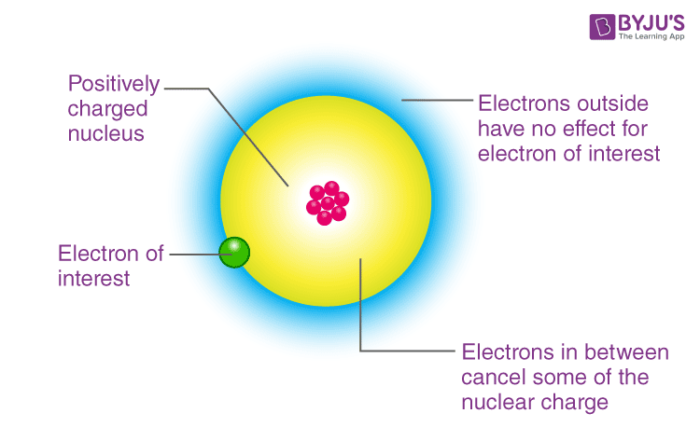# Electron Gain Enthalpy Of Elements In Modern Periodic Table

Electron gain enthalpy (ΔegH) is defined as the enthalpy change associated with an isolated gaseous atom (X) when it gains an electron to form its corresponding anion. The reaction can be given as below:

$$\begin{array}{l}X (g) + e^{-} \rightarrow X^{-} (g)\end{array}$$

On the basis of the nature of the element, the process of accepting electron in an atom can either be exothermic or endothermic. In general, energy is released when an electron is added to an atom and the electron gain enthalpy for such elements is negative. The electron gain enthalpy of halogens is highly negative because it needs only one electron to achieve the nearest noble gas configuration. And for noble gases, it is highly positive because the extra electron has to be placed in the next higher principal quantum level which requires lots of energy.When one electron is added to an atom, it becomes negatively charged ions. Now if we add the second electron to this negatively charged ion, it experiences repulsive forces due to the electrons already present in that shell. Additional energy should be provided to overcome these repulsive forces. Hence, the second electron gain enthalpy of an element is positive. Example: When an electron is added to sulphur atom to form S ion, it releases energy whereas energy is required when another electron is added to S ion to form S2- ion.

First electron gain enthalpy:

$$\begin{array}{l}S (g) + e^{-} \rightarrow S^{-}(g)\end{array}$$
;
$$\begin{array}{l}Δ_{eg}H_1 = -200 kJ mol{-1}\end{array}$$
(energy is released)

Second electron gain enthalpy:

$$\begin{array}{l}S^{-}(g) + e^{-}(g) \rightarrow S^{2-} (g)\end{array}$$
;
$$\begin{array}{l} Δ_{eg}H_2\end{array}$$
=
$$\begin{array}{l}+590 kJ mol^{-1}\end{array}$$
((energy is absorbed)

Factors responsible for variation:

1. Atomic size
2. Nuclear charge
3. Electronic configuration

Variation in electron gain enthalpy in the period: In the modern periodic table, on moving from left to right across a period, the atomic size of elements decreases and the effective nuclear charge increases. Thus, the force of attraction between the nucleus and added electron increases. Hence, electron gain enthalpy becomes more negative while moving right across a period.

Variation of electron gain enthalpy in a group: Generally, when we move down in a group becomes less negative. This is because, as we go down the group both the atomic size and nuclear charge increases, but the effect of atomic size is more prominent than that of nuclear charge. Hence, the force of attraction between the nucleus and the added electron decreases and thus enthalpy becomes less negative.

This was the general trend for electron gain enthalpy in a modern periodic table. To know more about exceptions in the variation and reasons behind them. Please subscribe to BYJU’S channel on YouTube.’

Test your knowledge on Electron Gain Enthalpy Of Elements In Modern Periodic Table